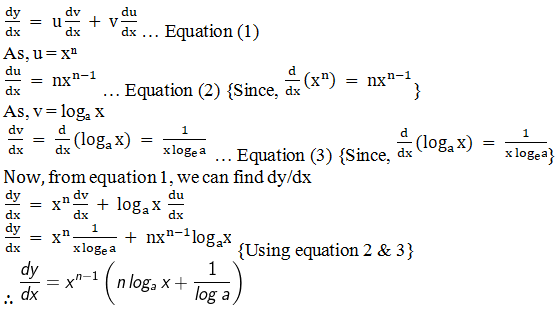# RD Sharma Solutions for Class 11 Chapter 30 - Derivatives Exercise 30.4

This exercise deals with fundamental rules for differentiation which is based on the product rule for differentiation. The solutions here are created by the expert faculty team at BYJU’S. Students can practice these problems in the most efficient ways and can also focus on cracking the solutions of Maths in such a way that it is easy for them to understand. RD Sharma Class 11 Maths Solutions mainly aims at equipping the students with detailed and step-wise explanations for all the answers to the questions given in the exercises of this Chapter. The pdf of RD Sharma Solutions can be downloaded from the links given below.

## Download the pdf of RD Sharma Solutions for Class 11 Maths Exercise 30.4 Chapter 30 – Derivatives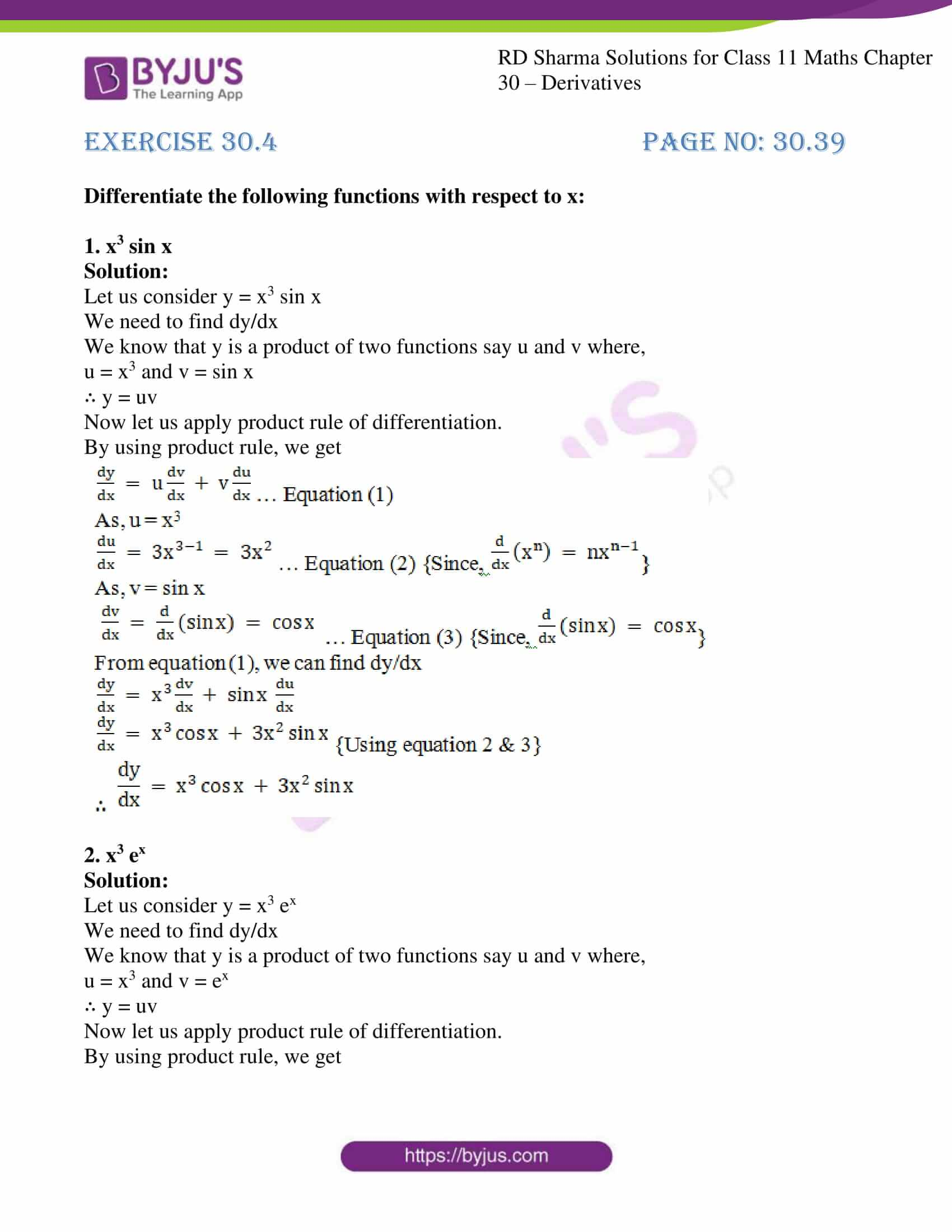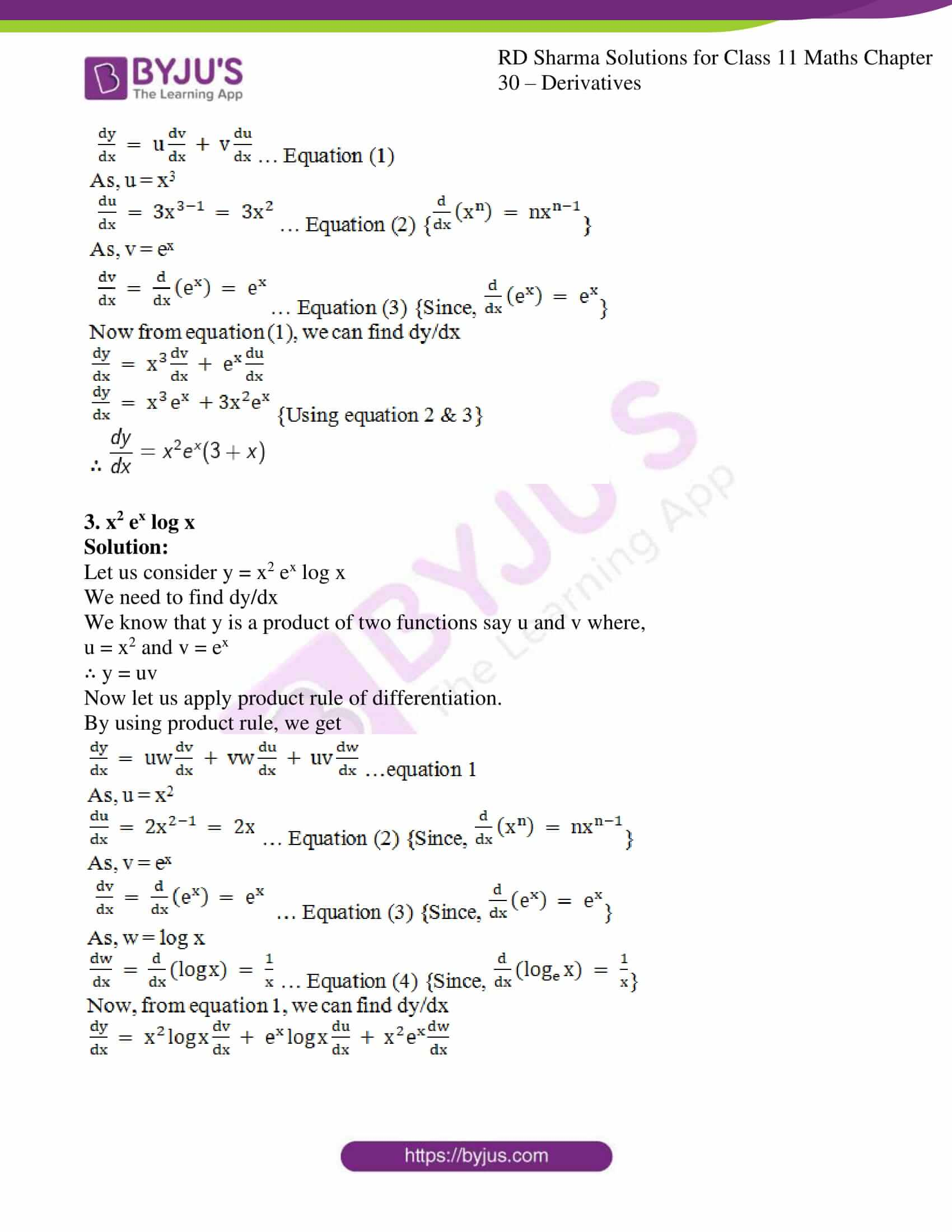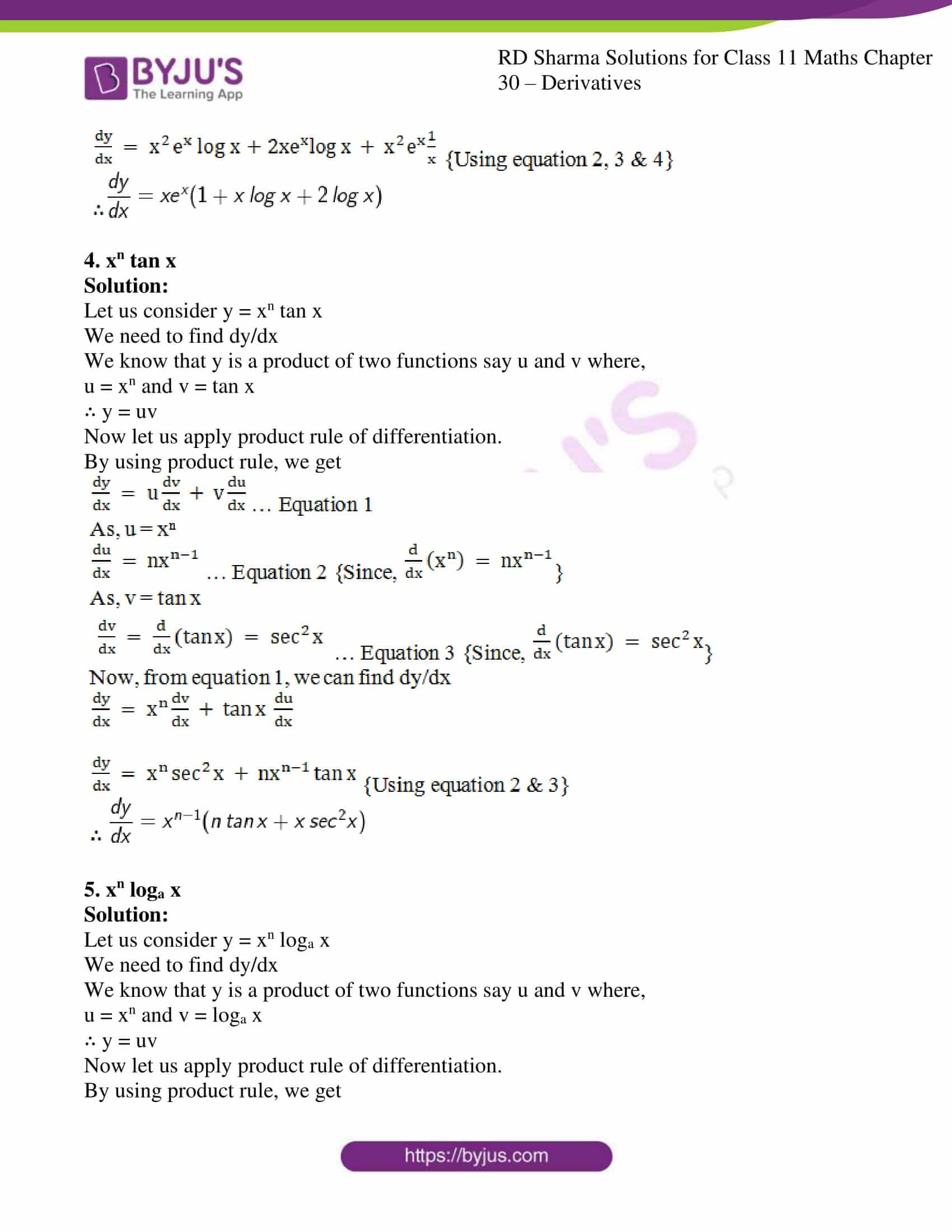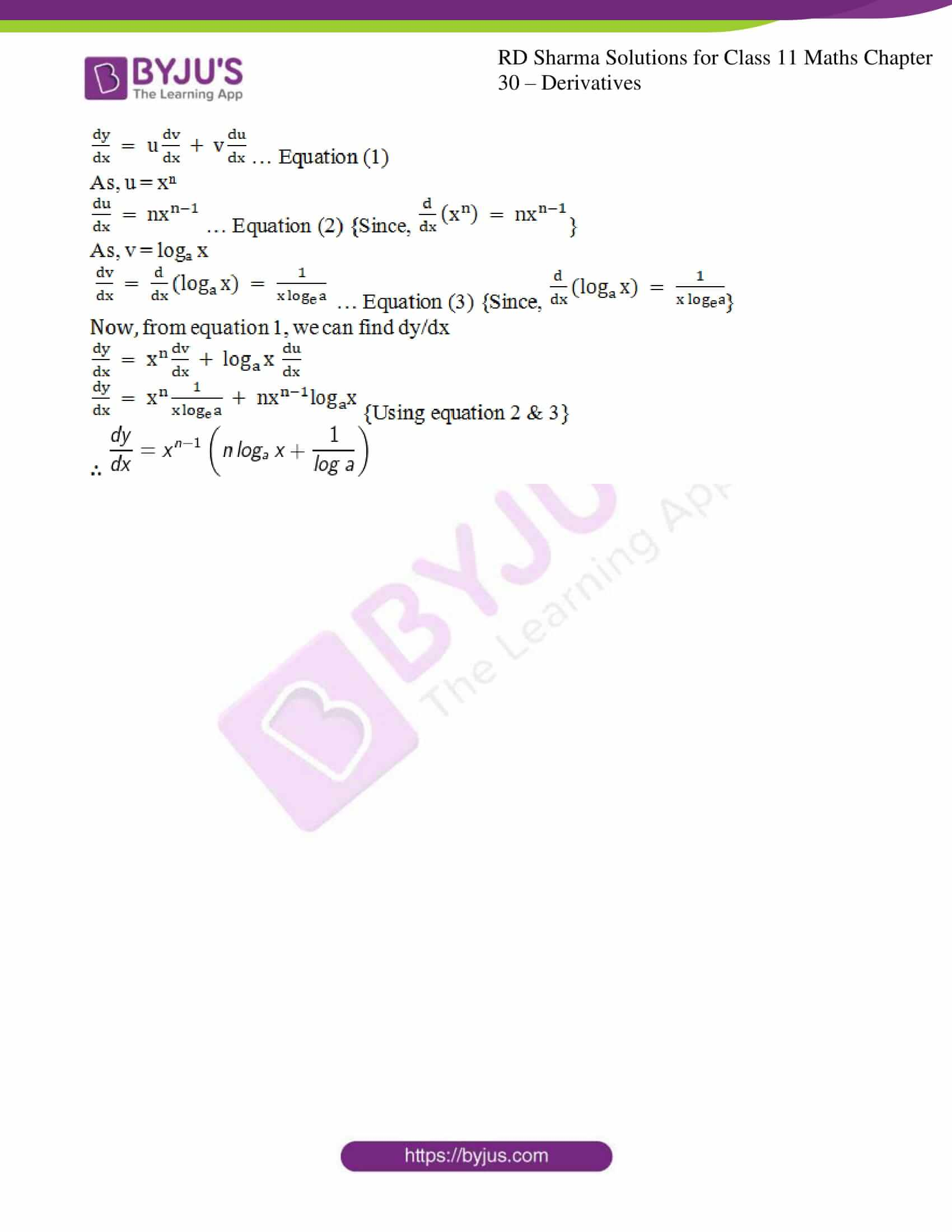### Also, access other exercises of RD Sharma Solutions for Class 11 Maths Chapter 30 – Derivatives

Exercise 30.1 Solutions

Exercise 30.2 Solutions

Exercise 30.3 Solutions

Exercise 30.5 Solutions

### Access answers to RD Sharma Solutions for Class 11 Maths Exercise 30.4 Chapter 30 – Derivatives

#### EXERCISE 30.4 PAGE NO: 30.39

Differentiate the following functions with respect to x:

1. x3 sin x

Solution:

Let us consider y = x3 sin x

We need to find dy/dx

We know that y is a product of two functions say u and v where,

u = x3 and v = sin x

∴ y = uv

Now let us apply product rule of differentiation.

By using product rule, we get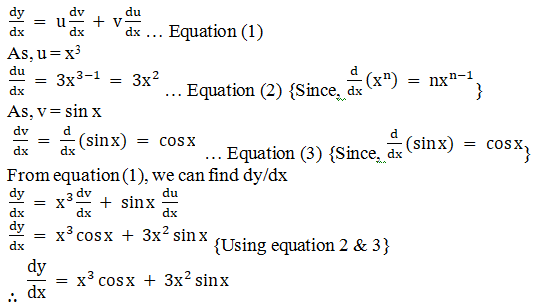2. x3 ex

Solution:

Let us consider y = x3 ex

We need to find dy/dx

We know that y is a product of two functions say u and v where,

u = x3 and v = ex

∴ y = uv

Now let us apply product rule of differentiation.

By using product rule, we get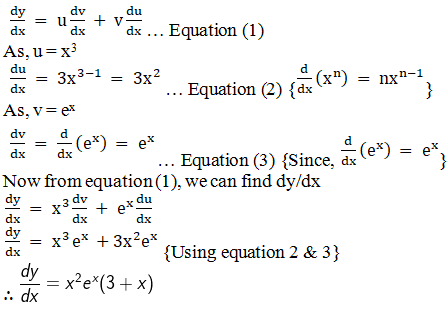3. x2 ex log x

Solution:

Let us consider y = x2 ex log x

We need to find dy/dx

We know that y is a product of two functions say u and v where,

u = x2 and v = ex

∴ y = uv

Now let us apply product rule of differentiation.

By using product rule, we get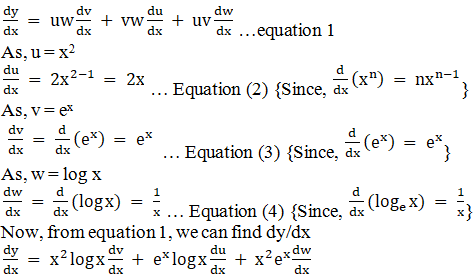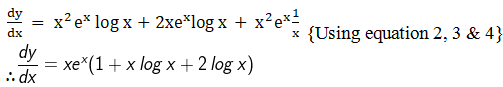4. xn tan x

Solution:

Let us consider y = xn tan x

We need to find dy/dx

We know that y is a product of two functions say u and v where,

u = xn and v = tan x

∴ y = uv

Now let us apply product rule of differentiation.

By using product rule, we get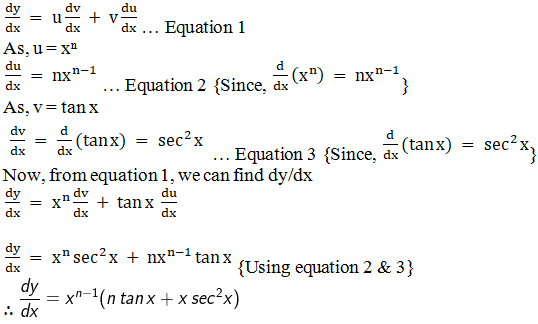5. xn loga x

Solution:

Let us consider y = xn loga x

We need to find dy/dx

We know that y is a product of two functions say u and v where,

u = xn and v = loga x

∴ y = uv

Now let us apply product rule of differentiation.

By using product rule, we get